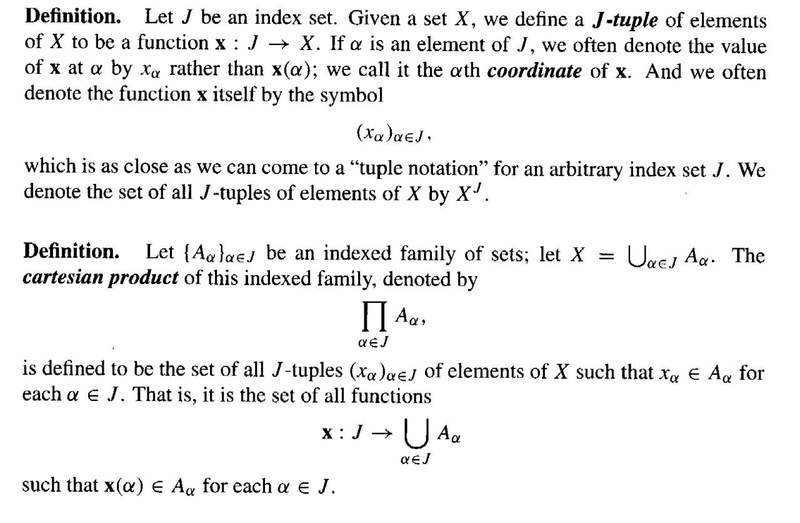# Simple set theory problem - definition of a J-Tuple (1 Viewer)

### Users Who Are Viewing This Thread (Users: 0, Guests: 1)

#### Math Amateur

On page 113 Munkres (Topology: Second Edition) defines a J-tuple as follows:I was somewhat perplexed when I tried to completely understand the function $\ x \ : \ J \to X$.

I tried to write down some specific and concrete examples but still could not see exactly how the function would work.

For example if $J = \{1, 2, 3 \}$ and X was just the collection of all the letters of the alphabet i.e.

$X = \{ a, b, c, ... \ ... \ z \}$ then ...

... obviously a map like 1 --> a, 2 --> d, 3 --> h does not work as the intention, I would imagine is to have a mapping which specifies a number of triples ... but how would this work?

Can someone either correct my example or give a specific concrete example that works.

Would appreciate some help.

Peter

#### micromass

Let's say $X=\mathbb{R}$. The usual definition of a $3$-tuple is just $(x_1,x_2,x_3)$ where each $x_i\in \mathbb{R}$. For example $(1,1,1)$ and $(2,1,2)$ are $3$-tuples.

This definition sees every $3$-tuple as a function $f:\{1,2,3\}\rightarrow \mathbb{R}$. Indeed, the $3$-tuple $(x_1,x_2,x_3)$ is presented as the function $f$ such that $f(k) = x_k$. So for example, the $3$-tuple $(1,1,1)$ is the constant function $f(k)=1$, while $(2,1,2)$ is presented by the function $f(1) = 2$, $f(2)=1$, $f(3) = 2$.

This generalizes beyond $\{1,2,3\}$ of course. If you're familiar with a sequence in $\mathbb{R}$, then you know that this is just a function $\mathbb{N}\rightarrow \mathbb{R}$. This is thus simply an $\mathbb{N}$-tuple.

•1 person

#### Math Amateur

Let's say $X=\mathbb{R}$. The usual definition of a $3$-tuple is just $(x_1,x_2,x_3)$ where each $x_i\in \mathbb{R}$. For example $(1,1,1)$ and $(2,1,2)$ are $3$-tuples.

This definition sees every $3$-tuple as a function $f:\{1,2,3\}\rightarrow \mathbb{R}$. Indeed, the $3$-tuple $(x_1,x_2,x_3)$ is presented as the function $f$ such that $f(k) = x_k$. So for example, the $3$-tuple $(1,1,1)$ is the constant function $f(k)=1$, while $(2,1,2)$ is presented by the function $f(1) = 2$, $f(2)=1$, $f(3) = 2$.

This generalizes beyond $\{1,2,3\}$ of course. If you're familiar with a sequence in $\mathbb{R}$, then you know that this is just a function $\mathbb{N}\rightarrow \mathbb{R}$. This is thus simply an $\mathbb{N}$-tuple.
Thanks micromass, been through your post carefully ... just to be sure ...

You write

"This definition sees every $3$-tuple as a function $f:\{1,2,3\}\rightarrow \mathbb{R}$."

... ... so in defining a set of triples or 3-tuples we are dealing with a set of functions, one function for every 3-tuple ... is that correct?

(Mind you I guess it is as Munkres was defining a (one) J-tuple ... rather than a function that described a set f J-tuples ,,,)

Peter

#### micromass

Thanks micromass, been through your post carefully ... just to be sure ...

You write

"This definition sees every $3$-tuple as a function $f:\{1,2,3\}\rightarrow \mathbb{R}$."

... ... so in defining a set of triples or 3-tuples we are dealing with a set of functions, one function for every 3-tuple ... is that correct?
One $3$-tuple can be seen as one function. So yes, a set of $3$-tuples can be seen as a set of functions this way.

(Mind you I guess it is as Munkres was defining a (one) J-tuple ... rather than a function that described a set f J-tuples ,,,)
Indeed, Munkres defined a $J$-tuple simply as a function.

•1 person

### The Physics Forums Way

We Value Quality
• Topics based on mainstream science
• Proper English grammar and spelling
We Value Civility
• Positive and compassionate attitudes
• Patience while debating
We Value Productivity
• Disciplined to remain on-topic
• Recognition of own weaknesses
• Solo and co-op problem solving